Next: Ray Tracing Up: Waves in Cold Plasmas Previous: Collisional Damping

# Pulse Propagation

Consider the situation under investigation in Sect. 4.12, in which a plane wave, polarized in the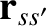-direction, is launched along the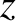-axis, from an antenna located at large positive, and reflected from a cutoff located at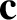. Up to now, we have only considered infinite wave-trains, characterized by a discrete frequency,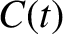. Let us now consider the more realistic case in which the antenna emits a finite pulse of radio waves.

The pulse structure is conveniently represented as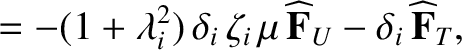(622)

where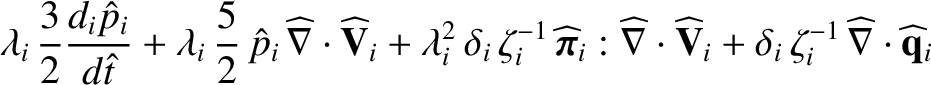is the electric field produced by the antenna, which is assumed to lie at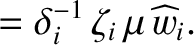. Suppose that the pulse is a signal of roughly constant (angular) frequency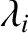, which lasts a time, whereis long compared to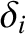. It follows that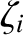possesses narrow maxima around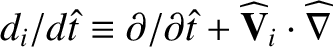. In other words, only those frequencies which lie very close to the central frequencyplay a significant role in the propagation of the pulse.

Each component frequency of the pulse yields a wave which propagates independently along the-axis, in a manner specified by the appropriate WKB solution [see Eqs. (569)-(570)]. Thus, if Eq. (622) specifies the signal at the antenna (i.e., at), then the signal at coordinate(where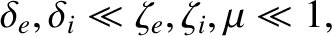) is given by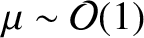(623)

where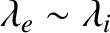(624)

Here, we have used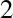.

Equation (623) can be regarded as a contour integral in-space. The quantity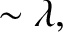is a relatively slowly varying function of, whereas the phase,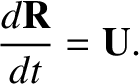, is a large and rapidly varying function of. The rapid oscillations of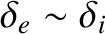over most of the path of integration ensure that the integrand averages almost to zero. However, this cancellation argument does not apply to places on the integration path where the phase is stationary: i.e., places where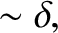has an extremum. The integral can, therefore, be estimated by finding those points wherehas a vanishing derivative, evaluating (approximately) the integral in the neighbourhood of each of these points, and summing the contributions. This procedure is called the method of stationary phase.

Suppose thathas a vanishing first derivative at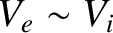. In the neighbourhood of this point,can be expanded as a Taylor series,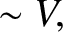(625)

Here, the subscript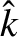is used to indicateor its second derivative evaluated at. Since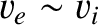is slowly varying, the contribution to the integral from this stationary phase point is approximately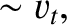(626)

The above expression can be written in the form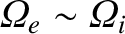(627)

where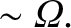(628)

The integrals in the above expression are Fresnel integrals,and can be shown to take the values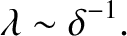(629)

It follows that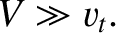(630)

If there is more than one point of stationary phase in the range of integration then the integral is approximated as a sum of terms like the above.

Integrals of the form (623) can be calculated exactly using the method of steepest decent.The stationary phase approximation (630) agrees with the leading term of the method of steepest decent (which is far more difficult to implement than the method of stationary phase) provided thatis real (i.e., provided that the stationary point lies on the real axis). Ifis complex, however, the stationary phase method can yield erroneous results.

It follows, from the above discussion, that the right-hand side of Eq. (623) averages to a very small value, expect for those special values ofand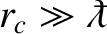at which one of the points of stationary phase in-space coincides with one of the peaks of. The locus of these special values ofandcan obviously be regarded as the equation of motion of the pulse as it propagates along the-axis. Thus, the equation of motion is specified by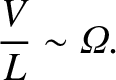(631)

which yields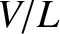(632)

Suppose that the-velocity of a pulse of central frequencyat coordinateis given by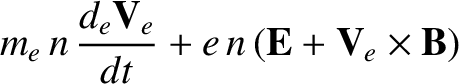. The differential equation of motion of the pulse is then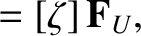. This can be integrated, using the boundary conditionat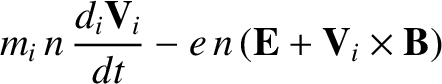, to give the full equation of motion: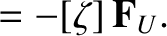(633)

A comparison of Eqs. (632) and (633) yields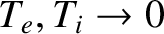(634)

The velocity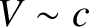is usually called the group velocity. It is easily demonstrated that the above expression for the group velocity is entirely consistent with that given previously [see Eq. (520)].

The dispersion relation for an electromagnetic plasma wave propagating through an unmagnetized plasma is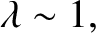(635)

Here, we have assumed that equilibrium quantities are functions ofonly, and that the wave propagates along the-axis. The phase velocity of waves of frequencypropagating along the-axis is given by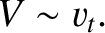(636)

According to Eqs. (634) and (635), the corresponding group velocity is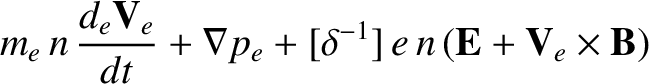(637)

It follows that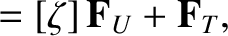(638)

It is assumed that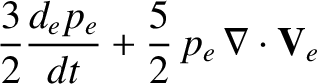, and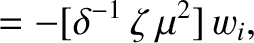for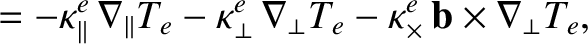, which implies that the reflection point corresponds to. Note that the phase velocity is always greater than the velocity of light in vacuum, whereas the group velocity is always less than this velocity. Note, also, that as the reflection point,, is approached from positive, the phase velocity tends to infinity, whereas the group velocity tends to zero.

Although we have only analyzed the motion of the pulse as it travels from the antenna to the reflection point, it is easily demonstrated that the speed of the reflected pulse at positionis the same as that of the incident pulse. In other words, the group velocities of pulses traveling in opposite directions are of equal magnitude.Next: Ray Tracing Up: Waves in Cold Plasmas Previous: Collisional Damping
Richard Fitzpatrick 2011-03-31